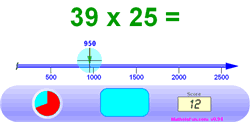# Estimation Tips and Tricks

Estimation is finding a number that is close enough to the right answer.

• You are not trying to get the exact right answer
• What you want is something that is good enough (usually in a hurry!)Example: you want to buy five magazines that cost \$1.95 each. When you go to buy them the cost is \$12.25. Is that right?

"five lots of \$1.95 is about 5 times 2, or about \$10"

so \$12.25 seems too much!

Ask to have the total checked.

Estimation helps with your Confidence, Judgement and Decisions!

## How to Estimate

Before doing the actual calculation you should think:

"how do I go about making this estimate?"

Because different numbers need different methods:

Example:

• 550 + 298: 298 is nearly 300 so an estimate is 550+300 = 850
• 550 + 248: 50+48 is nearly 100 so an estimate is 500+200 +100 = 800

In one case it seemed easy to change one number and then add.

In the other case I added the hundreds together and then increased the result by 100

There is no "right way",

Whatever works for you is fine!

But how do you know what to do? Lots of Practice!So we made some Estimation Games for you to practice with.

Together with the following Tips and Tricks, you will become a Master at Estimation.

## Tips and Tricks

Here are a few methods you might like to use:

Concentrate on the first digit of each number ... it will have the biggest impact on the answer. You can then look at the other digits to make small adjustments to your answer.

Example: 2156 + 3809.

Add 2000 and 3000 to get 5000. Then look at the rest of the numbers: "156 plus 809 is nearly a thousand", so increase your answer to 6000.

That also works with decimal numbers:

Example: what is 0.3126 times 53.81. Multiply 0.3 × 50 to get 15. Adjust that a little bit higher, and make your answer 17.

Round the numbers up or down before the calculation.

Example: 206 × 390.

Because 206 is nearly 200, and 390 is nearly 400, the answer will be close to

200 × 400 = 80,000

Check the number of zeros in your calculation!

In the previous example I calculated 200 × 400 = 80,000. How did I know how many zeros?

That was easy: after multiplying 2×4 to get 8, I took the two zeros from 200 plus the two zeros from 400, to make four zeros after the 8: 80000

When adding a lot of similar numbers, look at the numbers and choose an average, and then multiply that average by how many numbers

Example: What is 345 + 380 + 310 + 375 + 330 + 362?

There are 6 numbers, which seem to be around 350:

6 × 350 = 2100

When doing division, change the numbers to fit in with the multiplication tables

Example: what is 176 divided by 3?

Change 176 to 180 (because 3×6=18) and then do:

180 / 3 = 60

then adjust a little lower to 59

Group numbers together that will be easy to work on

Example: what is 76 + 49 + 22 + 53?

76 and 22 are nearly 100.

And 49 and 53 are also about 100.

Example: what is 52 × 13 × 20.

The two outer number, 52 and 20 multiply to be about 1000 (5×2=10),

THEN multiply by 13 to get 13,000

With decimals, percents and fractions try to think what the number means. Think: is it close to 1? Close to half? Close to zero?

Example: 1.6 × 30.

1.6 is close to 1.5, which is 1 and a half.

So 1.6 × 30 is close to 30 plus half of 30, which is 30 + 15 = 45.

Adjust a little higher for an estimate of 47

Example: 0.108 × 50.

0.108 is close to one-tenth, so 0.108 × 50 is close to one-tenth of 50 or about 5

Adjust a little higher for an estimate of 5.5

A percentage is like a decimal: 10% is 0.1, 50% is 0.5, etc.

Example: what is 20% of \$15?

20% is 0.2, or two-tenths.

One tenth of \$15 is \$1.50, so two tenths is \$3.00

Also, a fraction might be close to zero, close to half or close to one.

Example: what is 9/10 plus 7/8?

Both 9/10 and 7/8 are close to one, so the answer must be close to 2.

Example: what is 4/9 times 12?

4/9 is nearly half so the answer must be close to half of 12, or 6.

## Estimating Counts, Lengths and More

Estimation is not always about doing calculations! It is important for you to be able to estimate how many things you can see, or how long something is or how big something is.

See our page on Visual Estimation